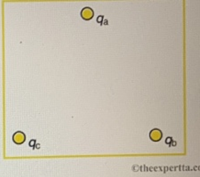# Problem: In the figure, the point charges are located at the corners of an equilateral triangle 21 cm on a side. Part (a) Find the magnitude of the electric field in N/C at the location of qa given that qb=10.8 uC and qc=4.8 uC. Part (b) Find the direction of the electric field at qa, in degrees above the negative x-axis with origin at qaPart (c) What is the magnitude of the force in N on qa given that qa=1.9 nC?Part (d) What is the direction of the force on qa, in degrees above the negative x-axis with origin at qa?

###### FREE Expert Solution

Electric field:

$\overline{){\mathbf{E}}{\mathbf{=}}\frac{\mathbf{k}\mathbf{q}}{{\mathbf{r}}^{\mathbf{2}}}}$

Part (a)

The electric field at qa due to qb is:

$\begin{array}{rcl}{\mathbf{E}}_{\mathbf{a}\mathbf{b}}& \mathbf{=}& \frac{\mathbf{\left(}\mathbf{9}\mathbf{×}{\mathbf{10}}^{\mathbf{9}}\mathbf{\right)}\mathbf{\left(}\mathbf{10}\mathbf{.}\mathbf{8}\mathbf{×}{\mathbf{10}}^{\mathbf{-}\mathbf{6}}\mathbf{\right)}}{{\mathbf{\left(}\mathbf{21}\mathbf{×}\mathbf{10}\mathbf{-}\mathbf{2}\mathbf{\right)}}^{\mathbf{2}}}\end{array}$

Eab = 2.204 × 106 N/C

The electric field at qa due to qc is:

$\begin{array}{rcl}{\mathbf{E}}_{\mathbf{ac}}& \mathbf{=}& \frac{\mathbf{\left(}\mathbf{9}\mathbf{×}{\mathbf{10}}^{\mathbf{9}}\mathbf{\right)}\mathbf{\left(}\mathbf{4}\mathbf{.}\mathbf{8}\mathbf{×}{\mathbf{10}}^{\mathbf{-}\mathbf{6}}\mathbf{\right)}}{{\mathbf{\left(}\mathbf{21}\mathbf{×}{\mathbf{10}}^{\mathbf{-}\mathbf{2}}\mathbf{\right)}}^{\mathbf{2}}}\end{array}$

Eac = 9.80 × 105 N/C###### Problem Details

In the figure, the point charges are located at the corners of an equilateral triangle 21 cm on a side.Part (a) Find the magnitude of the electric field in N/C at the location of qa given that qb=10.8 uC and qc=4.8 uC.

Part (b) Find the direction of the electric field at qa, in degrees above the negative x-axis with origin at qa

Part (c) What is the magnitude of the force in N on qa given that qa=1.9 nC?

Part (d) What is the direction of the force on qa, in degrees above the negative x-axis with origin at qa?